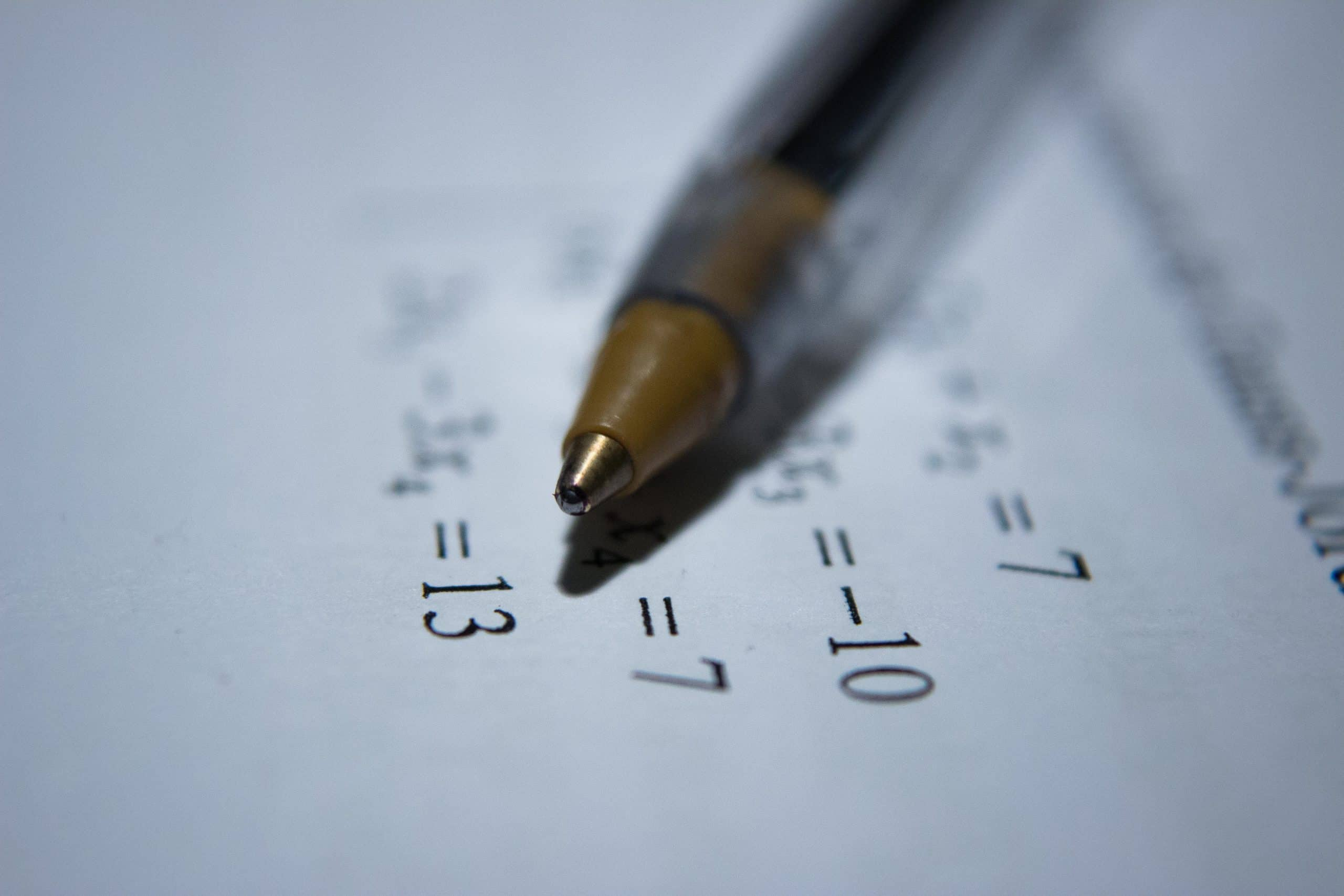Benefits of Learning Multiplication Tables from 1 to 20

Multiplication, apart from addition, subtraction and division, forms one of the four basic mathematical operations. It may not be an easy concept to grab for many, but it’s a lifesaver for mathematics problem-solving. The multiplication chart, thus, constitutes an integral part of calculations. Without encountering multiplication in everyday life, we cannot go by a single day, and even the daily simple calculations cannot go untouched by multiplication.Suppose you go to a zoo with three of your friends, and for that, you need to purchase tickets. If the expense of one ticket is known to you, the total price charged for all of you can be accurately determined. If you know the table of four, you just need to multiply four by the ticket price. Another example, you go to the shop, to calculate the number of items to be paid you need to know multiplication. Multiplication has its presence everywhere from the measurements of ingredients in the kitchen to prepare food to the stock markets.

Multiplication is also referred to as a repetitive addition because as quoted in the above example of a zoo ticket, you multiply the ticket cost by four and pay the sum instead of adding the ticket’s price four times. That’s the advantage of multiplication. It saves the time needed for the calculation. It is essential to demonstrate how addition forms the building block of multiplication before explaining multiplication to children. Tables 1 to 20 play an important role in math. There are various methods and strategies to learn these tables quickly. If the child is finding the higher number of tables challenging to memorize. The main point here being the concept of multiplication should be clear.

If you are like me and have some difficulties with Maths and learn a bit more easily if you are having fun, or if you want to get the little ones a bit more engaged and thriving in Maths at school, you should try multiplication games. There are a lot of online tools and websites on this topic and I find it much easier to assimilate topics that I struggle with if I am having some fun in the process. These method is great to learn multiplication tables no matter what grade you are in.

If practiced for even a few minutes regularly, and if the multiplication rules are clear to the child, then arithmetic usually becomes the favorite subject of the child. Multiplication tables are usually dreaded as there are a lot of numbers to be dealt with, and most tables are viewed as something that can only be mastered by memorizing over and over again. Things can be made simpler if children are taught counting by using illustrations or by showing them those grouping things aids in quicker calculations or making groups of toys of the same number will help them count properly.

People who are experts at calculations who are able to solve problems quickly are not inherently intelligent, and it is because they have learned the art of arithmetic that helps them to do mental math at a faster speed. In general, people perceive quick mathematical abilities as high intelligence, but it is mostly due to these few tricks that help in the long term when well practiced. To find multiplication worksheets with tips and tricks for problem-solving, visit the Cuemath website.

The benefits of learning multiplication tables from 1 to 20 can assist kids in making fast estimates that can have long-term advantages. If the kid is good at crunching numbers, it would not only help them in their school studies but in daily life as well. Multiplication is involved in higher math topics such as arithmetic, geometry, calculus. Math, as we know, is a number manipulation game, and one has to be knowledgeable in mathematics to understand the mechanism to understand these manipulations. And multiplication forms one of the core things to be understood well.

Teachers need to concentrate on developing a proven method of teaching that will help kids to learn multiplication tables and solve problems in less time. Learning to multiply can also help children figure out how fun it would be to play with numbers. This would result in improved analytical skills, increased trust, and self-esteem, discovering new problem-solving methods and tactics, and setting themselves up for more math progress.

Many of the beautiful natural patterns such as binomial series and factorials are identified by multiplication operation, which led to the development of many useful theorems that benefited civilization.